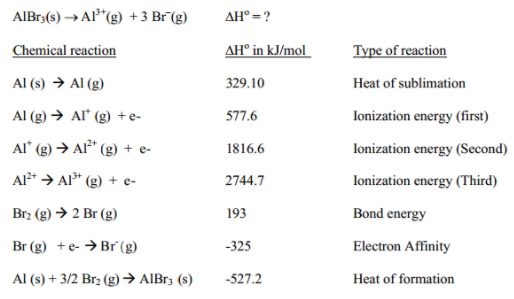Problem: Calculate lattice energy. The lattice energy of an ionic compound is the energy change when one mole of ionic solid is separated into its gaseous ions. Given the data below, find lattice energy for AlBr3, which is the ΔH° for the following reaction:

FREE Expert Solution
79% (192 ratings)
Problem Details

Calculate lattice energy. The lattice energy of an ionic compound is the energy change when one mole of ionic solid is separated into its gaseous ions. Given the data below, find lattice energy for AlBr3, which is the ΔH° for the following reaction:What scientific concept do you need to know in order to solve this problem?

Our tutors have indicated that to solve this problem you will need to apply the Born Haber Cycle concept. You can view video lessons to learn Born Haber Cycle Or if you need more Born Haber Cycle practice, you can also practice Born Haber Cycle practice problems .

What is the difficulty of this problem?

Our tutors rated the difficulty of Calculate lattice energy. The lattice energy of an ionic com... as high difficulty.

How long does this problem take to solve?

Our expert Chemistry tutor, Dasha took 12 minutes to solve this problem. You can follow their steps in the video explanation above.

What professor is this problem relevant for?

Based on our data, we think this problem is relevant for Professor Claridge & Ramachandran's class at PURDUE.